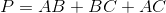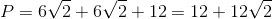## Example Questions

### Example Question #2 : How To Find The Perimeter Of A Right Triangle

Three points in the xy-coordinate system form a triangle.

The points are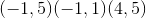.

What is the perimeter of the triangle?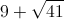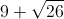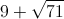Explanation:

Drawing points gives sides of a right triangle of 4, 5, and an unknown hypotenuse.

Using the pythagorean theorem we find that the hypotenuse is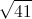.

### Example Question #5 : How To Find The Perimeter Of A Right Triangle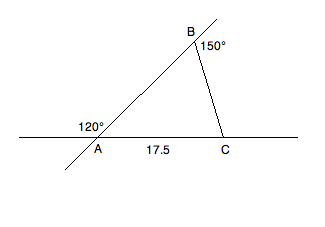Based on the information given above, what is the perimeter of triangle ABC?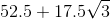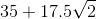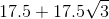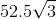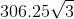Explanation: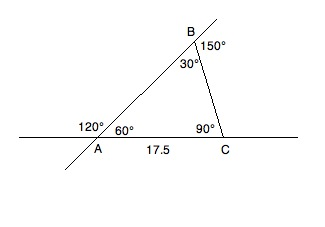Consult the diagram above while reading the solution. Because of what we know about supplementary angles, we can fill in the inner values of the triangle. Angles A and B can be found by the following reductions:

A + 120 = 180; A = 60

B + 150 = 180; B = 30

Since we know A + B + C = 180 and have the values of A and B, we know:

60 + 30 + C = 180; C = 90

This gives us a 30:60:90 triangle. Now, since 17.5 is across from the 30° angle, we know that the other two sides will have to be √3 and 2 times 17.5; therefore, our perimeter will be as follows: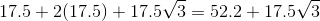### Example Question #1 : How To Find The Perimeter Of A Right Triangle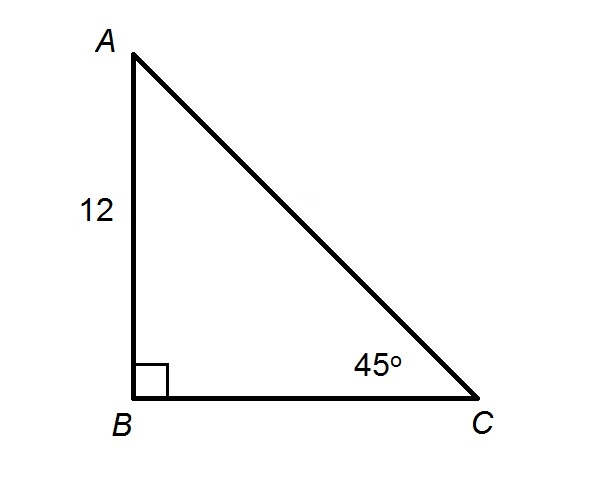Give the perimeter of the provided triangle.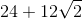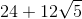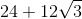Explanation:

The figure shows a right triangle. The acute angles of a right triangle have measures whose sum is, so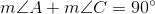Substituting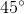for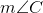: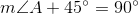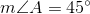This makes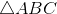a 45-45-90 triangle.

By the 45-45-90 Triangle Theorem, legs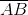and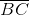are of the same length, so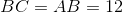.

Also by the 45-45-90 Triangle Theorem, the length of hypotenuse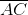is equal to that of legmultiplied by. Therefore,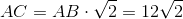.

The perimeter of the triangle is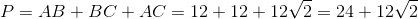### Example Question #2 : How To Find The Perimeter Of A Right Triangle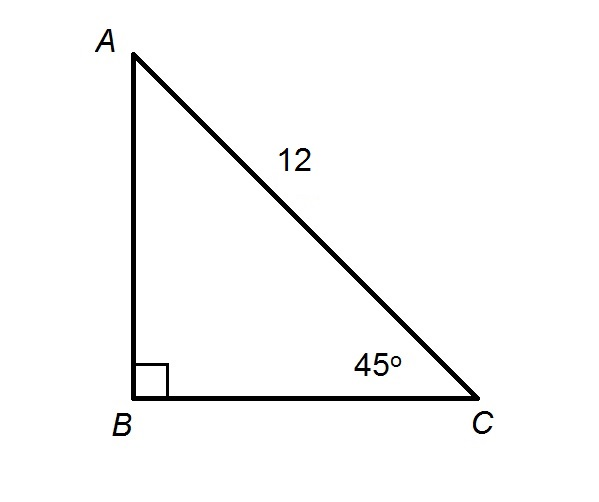What is the perimeter of the triangle above?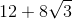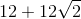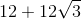Explanation:

The figure shows a right triangle. The acute angles of a right triangle have measures whose sum is, soSubstitutingfor:This makesa 45-45-90 triangle. By the 45-45-90 Triangle Theorem, the length of legis equal to that of hypotenuse, the length of which is 12, divided by. Therefore,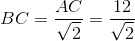Rationalize the denominator by multiplying both halves of the fraction by: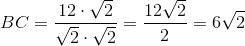By the same reasoning,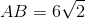.

The perimeter of the triangle is